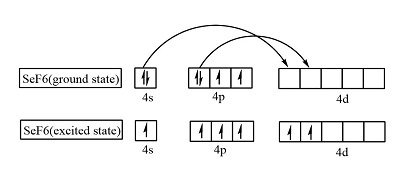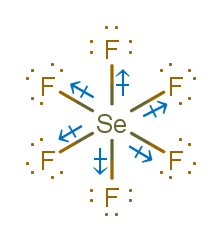# SeF6 Lewis Structure, Geometry, Hybridization, and PolaritySelenium hexafluoride is an inorganic compound composed of Selenium and fluorine atoms.

It is a colorless, toxic, and corrosive gas with a characteristic odor.

In this article, we will discuss essential concepts required for drawing Lewis structure and predicting geometry, hybridization, and polarity for a given compound.

Contents

## Lewis Structure

Lewis structure is a structural formula for representing the arrangement of valence shell electrons around each atom in a molecule.

It is important for predicting the number and type of bonds formed by an atom.

In a Lewis structure, electrons are represented by dots, and bonds are represented by lines around the chemical symbol of an element. Two dots on adjacent atoms are joined together to form a bond.

Some compounds can have more than one possible lewis dot structure. In such cases, the one that satisfies the octet rule and has the lowest number of formal charges is the most stable.## Octet Rule

Main group elements prefer to have an electronic configuration similar to noble gases as they are considered to be inert.

Noble gases have 8 valence electrons (except He), and all other main group elements lose or gain electrons to achieve this configuration.

Thus, the preference of having 8 electrons in the valence shell of main group elements is called the octet rule.

It is one of the primitive concepts. Many compounds are stable but do not follow the octet rule. Exceptions to octet rule are-

• Odd electron species can never complete their octet because the sum of electrons is always odd. For example, NO, NO2, etc.

Hypo valent Species– some species have less than 8 electrons in their valence shell and are still stable. For example, B in BH3, Al in AlCl3, etc.

Hypervalent Species– some species have more than 8 electrons in their valence shell and are still stable. The atoms with more than 2 shells can expand their octet by including vacant d orbitals. For example, S in SF6, etc.

## Formal Charge

It gives a direct relationship between the number of electrons on an isolated neutral atom and the number of electrons present on that atom when it is present in a combined form.

Formal charge= (number of electrons in an isolated neutral atom)- (number of nonbonding electrons on an atom in the compound)- 0.5*(number of electrons shared in bonds by atom)

For the lowest formal charge, one must have a zero formal charge on as many atoms as possible.

If a formal charge is present, a negative formal charge should be on more electronegative elements, while a positive formal charge should be on the less electronegative element.

## Steps to Draw SeF6 Lewis Structure

Step 1: Count the total number of valence shell electrons on the compound

Before drawing the structure, we need to know the number of valence shell electrons on all constituent atoms and their sum.Step 2: Draw the lewis dot structure for elements.

We draw the Lewis structure of elements by arranging the valence shell electrons around the element’s chemical symbol.

The chemical symbols for Selenium and fluorine are Se and F, respectively. The Lewis dot structure for Se and F are as follows-Step 3: Choose a suitable central atom for the compound.

The central atom is supposed to be the least electronegative one out of the constituent atoms, as the central atom is supposed to share its electron density with all other atoms.

Thus, Se is the central atom for this compound.

Step 4: Draw a skeletal diagram.

In this step, we have to arrange the side atoms and central atom suitably.Step 5: Arrange the valence electrons around the elemental symbols.

The total valence shell electrons (calculated in step 1) are placed around the symbol of chemical elements. Orange dots represent electrons of Selenium, while black dots represent electrons of fluorine.Step 6: Complete the octet of atoms by forming bonds.

All side atoms form a single bond with the central atom.Each F has seven valence electrons in the isolated state. They share one electron with Se to have a fully filled valence shell configuration.

Se has six valence electrons in the isolated state. It shares one electron from all F-atoms.

There are 12 electrons around the central atom. SeF6 is a hypervalent compound as Se can expand its octet because of vacant d orbitals and form more than four bonds.

Step 7: Calculate the formal charge on all atoms.

The net charge on this compound is zero. Therefore, the sum of formal charge on seven atoms should come out to be zero.Thus, the structure drawn in step 6 is the best Lewis structure for SeF6.

To understand the process of drawing lewis structure better, one can go through this link.

## SeF6 Geometry

Molecular geometry is the 3D arrangement of atoms in a compound. Exact geometry can be found out only by experimentation in the laboratory.

However, we can use VSEPR theory to predict the shape without experimentation. VSEPR theory stands for valence electron pair repulsion theory.

According to VSEPR theory-

The valence electron pairs repel each other, and this leads to instability.

To make the arrangement of the electrons stable, the repulsions between them have to be decreased.

As a result, electrons align themselves so that the repulsion is the least, and the distance between them is maximum.

The stable arrangement of the valence electron pairs of atoms helps determine the molecular geometry.

Valence shell electrons involved in bonding are known as bonding pairs of electrons (bp), and those valence shell electrons that are not involved in bonding are termed as lone pairs of electrons (lp).

### How to Predict Geometry of SeF6 Using VSEPR

Step 1: Count the number of valence shell electrons on the central atom and let it be equal to A (arbitrary variable)

In the case of SeF6, the central atom is Se. Se has 6 valence electrons. (Shown in step1 of drawing lewis structure)

A=6

Step 2: Count the number of side atoms and let it be equal to B (arbitrary variable).

In SeF6, there are six side atoms (fluorine) and B=6

Step 3: If the compound is charged, subtract the charge from B for the positively charged compound and add the charge to B for the negatively charged compound. This step can be avoided for neutral compounds.

In SeF6, there is no contribution of charge and B=6 only.

Step 4: Add the contribution of side atoms and charge to the contribution of the central atom, i.e., A+B

For SeF6, A+B=12

Step 5: Divide A+B by 2 to find total electron pairs affecting the shape.

For SeF6, there are 6 electron pairs.

Step 6: Divide the total electron pairs as bonding and non-bonding. The bonding electron pair is equal to the number of side atoms.

For SeF6, there are six side atoms. Thus, there are six bonding pairs of electrons and zero nonbonding pairs of electrons.

Using this information, we can predict geometry and shape using the following table.

Step 7: Electron geometry and shape are octahedral. The geometry and shape are the same for compounds with zero lone pairs.SF6 is a very similar compound. Readout SF6 Lewis Structure, Geometry, hybridization, and Polarity.

## SeF6 Hybridization

Hybridization is one of the fundamental concepts used to explain bond formation. Pauling introduced it.

Hybridization involves mixing two or more atomic orbitals to form orbitals with the same energy, shape, and size. The hybridized orbitals form bonds, and the extent of overlap is better than unhybridized orbitals.

It does not involve literal mixing. Just mixing of wavefunctions takes place.

For example, one 4s and one 4p can form two sp hybrid orbitals, but 1s and 5p cannot.

In SeF6, Se is the central atom. We are focusing on the hybridization of the central atom only.

In the ground state, Se has 2 unpaired electrons. It can only form two bonds.

Promotion of electrons takes place, and all 6 valence electrons become unpaired.

These 6 electrons are present in different orbitals. All the 6 orbitals undergo hybridization to form sp3d2 hybrid orbitals.### The trick for calculating the type of hybridization of SeF6

In VSEPR theory, we calculated the total electron pairs in the last step.

For SeF6, it came out to be 6.

The table below can predict hybridization using total electron pairs or steric numbers.

Steric number = number of (sigma bonds +lone pair on central atom)

Steric number for SeF6= (6+0)=6

Hybridization comes out to be sp3d2 from the table.## SeF6 Polarity

The polarity of a compound depends on the presence or absence of the net dipole moment of a compound.

The net dipole moment depends on

1. The dipole moment of bonds
2. The electronegativity difference between atoms
3. Geometry

In SeF6, only one type of bond is present ie; Se-F bond. The electronegativity of Se and F is 2.55 and 3.98, respectively. The difference comes out to be 1.43. The bonds are polar, and the bond dipole moment is non-zero.

Polar bonds do not guarantee a polar compound. Dipole moment is a vector quantity. A symmetrical shape results in the cancellation of the dipole moment.The shape of SeF6 is octahedral. All the dipole moments are canceled, resulting in zero net dipole moment. Thus, the compound is non-polar.

Check out the article on SeF4 Polarity.

## Conclusion

SeF6 is a hypervalent compound.

The Lewis structure drawn in the above section is the most stable.

The shape and geometry for selenium hexafluoride are octahedral.

Hybridization of SeF6 is sp3d2.

It is a non-polar compound.